Maths-
General
Easy

Question

# If 5 men or 9 women can do a piece of work in 19 days then in how many days will 3 men and 6 women do the same work?Hint:

## The correct answer is: 15 days

### Step 1 of 3:It is given that 5 men can do a piece of work in 19 days and 9 women can do the same piece of work in 19 days. Let’s say the total work done is W and let’s say that the work done by one man is x and the work done by one woman is y. So we can say that5x = W ……..(1)and 9y = W ………(2)Equating equations (1) and (2)5x = 9yy =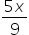………(3)Step 2 of 3:Now we are asked to determine the days that will be taken by 3 men and 6 women to do the same work. So we can say that3x + 6y = WFrom equation (3)3x + 6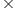= W3x +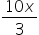= W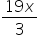= WSo, we can say that we need to find the number of days taken by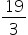men to complete the workStep 3 of 3:Let the number of days be represented as x and the number of men be represented as y. The number of men will increase if the number of days are decreased so we can conclude that y is in inverse relationship with x. Let’s say the proportional relationship is given asy =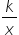……..(4)We now that 5 man can do a piece of work in 19 days i.e x = 19 and y = 5. Putting the values in equation (4)5 =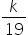k = 95We need to find the no. of days taken bymen. So,=Put the value of k = 95=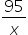x = 15 daysFinal Answer: Hence, 15 days are taken by 3 men and 6 women to do the same work.#### With Turito Foundation.#### Get an Expert Advice From Turito.